# Question Bank Solutions for SSC (English Medium) 8th Standard - Maharashtra State Board - Mathematics

Subjects
Topics
Subjects
Popular subjects
Topics
Mathematics
< prev  1 to 20 of 360  next >

In the adjoining figure, each angle is shown by a letter. Fill in the boxes with the help of the figure.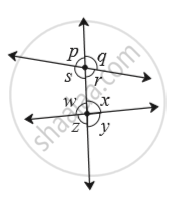Corresponding angles.

(1) ∠p and  ____
(2) ∠q and  ____
(3) ∠r and  ____
(4) ∠ s and _____ Interior alternate angles.
(5) ∠s and  _____
(6) ∠w and _____

[0.02] Parallel Lines and Transversal
Chapter: [0.02] Parallel Lines and Transversal
Concept: Pairs of Lines - Transversal

Observe the angles shown in the figure and write the following pair of angle.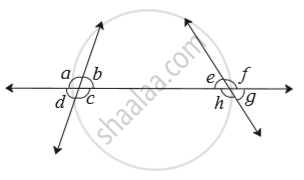Interior alternate angles

[0.02] Parallel Lines and Transversal
Chapter: [0.02] Parallel Lines and Transversal
Concept: Pairs of Lines - Transversal

Observe the angles shown in the figure and write the following pair of angle.Corresponding angles

[0.02] Parallel Lines and Transversal
Chapter: [0.02] Parallel Lines and Transversal
Concept: Pairs of Lines - Transversal

Observe the angles shown in the figure and write the following pair of angle.Interior angles

[0.02] Parallel Lines and Transversal
Chapter: [0.02] Parallel Lines and Transversal
Concept: Pairs of Lines - Transversal

Choose the correct alternative.

In the adjoining figure, if line m || line n and line p is a transversal then find x.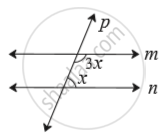[0.02] Parallel Lines and Transversal
Chapter: [0.02] Parallel Lines and Transversal
Concept: Pairs of Lines - Transversal

Choose the correct alternative.

In the adjoining figure, if line a || line b and line l is a transversal then find x.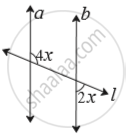[0.02] Parallel Lines and Transversal
Chapter: [0.02] Parallel Lines and Transversal
Concept: Pairs of Lines - Transversal

In the adjoining figure line p || line q.  Line t and line s are transversals. Find measure of ∠x and ∠y using the measures of angles given in the figure.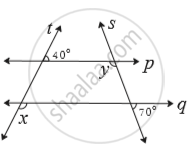[0.02] Parallel Lines and Transversal
Chapter: [0.02] Parallel Lines and Transversal
Concept: Pairs of Lines - Transversal

Express the following number in index form.

Fifth root of 13

[0.03] Indices and Cube Root
Chapter: [0.03] Indices and Cube Root
Concept: Concept of Exponents

Express the following number in index form.

Sixth root of 9

[0.03] Indices and Cube Root
Chapter: [0.03] Indices and Cube Root
Concept: Concept of Exponents

Express the following number in index form.

Square root of 256

[0.03] Indices and Cube Root
Chapter: [0.03] Indices and Cube Root
Concept: Concept of Exponents

Express the following number in index form.

Cube root of 17

[0.03] Indices and Cube Root
Chapter: [0.03] Indices and Cube Root
Concept: Concept of Exponents

Express the following number in index form.

Eighth root of 100

[0.03] Indices and Cube Root
Chapter: [0.03] Indices and Cube Root
Concept: Concept of Exponents

Express the following number in index form.

Seventh root of 30

[0.03] Indices and Cube Root
Chapter: [0.03] Indices and Cube Root
Concept: Concept of Exponents

In  LMN, _____ is an altitude and _____ is a median. (write the names of appropriate segments.)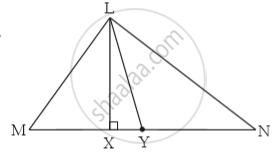[0.04] Altitudes and Medians of a Triangle
Chapter: [0.04] Altitudes and Medians of a Triangle
Concept: Altitudes of a Triangle

Draw an acute angled Δ PQR. Draw all of its altitudes. Name the point of concurrence as ‘O’.

[0.04] Altitudes and Medians of a Triangle
Chapter: [0.04] Altitudes and Medians of a Triangle
Concept: Altitudes of a Triangle

Draw a right angled Δ XYZ. Draw its medians and show their point of concurrence by G.

[0.04] Altitudes and Medians of a Triangle
Chapter: [0.04] Altitudes and Medians of a Triangle
Concept: Altitudes of a Triangle

Fill in the blanks.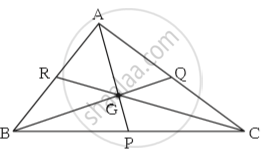Point G is the centroid of  ABC.
(1) If l(RG) = 2.5 then l(GC) = _____
(2) If l(BG) = 6 then l(BQ) = _____
(3) If l(AP) = 6 then l(AG) = _____   and l(GP) = _____

[0.04] Altitudes and Medians of a Triangle
Chapter: [0.04] Altitudes and Medians of a Triangle
Concept: Altitudes of a Triangle

Show the following numbers on a number line. Draw a separate number line for each example.

(1)3/2 , 5/2 , -3/2

(2)7/5 , (-2)/5 , (-4)/5

(3) (-5)/8 , 11/8

(4)13/10 , (-17)/10

[0.01] Rational and Irrational Numbers
Chapter: [0.01] Rational and Irrational Numbers
Concept: Rational Numbers on a Number Line

Observe the number line and answer the questions.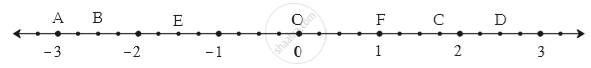(1) Which number is indicated by point B?

(2) Which point indicates the number 1 3/4 ?

(3) State whether the statement, ‘the point D denotes the number 5/2’ is true or false.

[0.01] Rational and Irrational Numbers
Chapter: [0.01] Rational and Irrational Numbers
Concept: Rational Numbers on a Number Line

Show the following numbers on a number line. Draw a separate number line for each example.

3/2 , 5/2 , -3/2

[0.01] Rational and Irrational Numbers
Chapter: [0.01] Rational and Irrational Numbers
Concept: Rational Numbers on a Number Line
< prev  1 to 20 of 360  next >